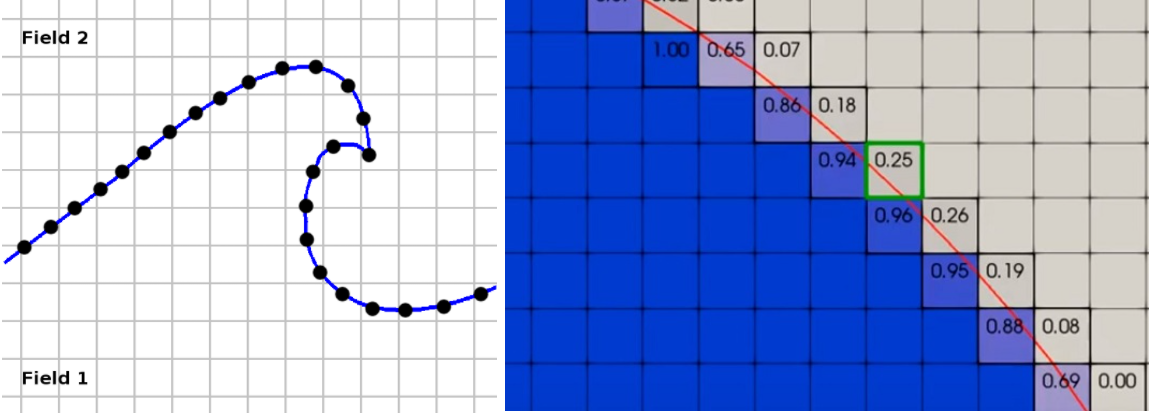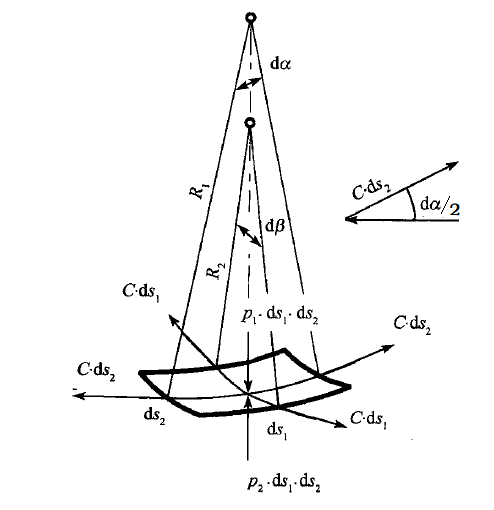# interFoam解析

## 引言

OpenFOAM中的VOF模型求解器，interFoam，可用于求解多相流的界面问题，如崩塌的堤坝、极少量的气泡界面、液体晃荡等。界面类存在两个关键问题：

• 界面尽可能要薄；

• 在界面失稳的情况下算法要尽可能的稳定；

(1)$\frac{\partial \rho \mathbf{U}}{\partial t}+\nabla \cdot (\rho \mathbf{U} \mathbf{U} ) - \nabla \cdot \tau =-\nabla p+\rho\bfg+\bfF$

(2)$\frac{\partial \rho}{\partial t}+\nabla \cdot (\rho \mathbf{U})=0$

(3)$\frac{\rD \rho}{\rD t}=\frac{\partial \rho}{\partial t}+\bfU\cdot\nabla\rho=0$

(4)$\nabla\cdot\bfU=0$## 控制方程(5)$F_p=(p_1 - p_2) \mathrm{d}s_1 \mathrm{d}s_2$

(6)$\begin{split} F_\sigma&=\sigma(\rd s_1 \rd\beta+\rd s_2\rd\alpha) =\sigma\left(\frac{\rd\beta}{\rd s_2}+\frac{\rd\alpha}{\rd s_1}\right)\rd s_1\rd s_2 \end{split}$

(7)$\frac{n_x|_{x+\Delta x/2}-n_x|_{x}}{\Delta x/2} \frac{|\Delta x|}{2}=\frac{\p n_x}{\p x} \frac{\rd x}{2}= \mathrm{sin}\frac{\alpha}{2} \approx \frac{\rd \alpha}{2}$

(8)$\frac{\p n_x}{\partial x} = \frac{\mathrm{d}\alpha}{\mathrm{d} x} = \frac{1}{R_1}$

(9)$\frac{\p n_y}{\partial y} = \frac{\mathrm{d}\beta}{\mathrm{d} y} = \frac{1}{R_2}$

(10)$\frac{\p n_z}{\partial z} = 0$

(11)$\begin{split} F_\sigma =\sigma\left( \frac{1}{R_1}+ \frac{1}{R_2} \right)\rd s_1\rd s_2 \end{split}$

(12)$\nabla \cdot \bfn = \frac{\partial \bfn}{\partial x} + \frac{\partial \bfn}{\partial y} + \frac{\partial \bfn}{\partial z} =\left( \frac{1}{R_1}+ \frac{1}{R_2} \right)$

(13)$\begin{split} F_\sigma =\sigma\nabla\cdot\bfn\rd s_1\rd s_2 \end{split}$

(14)$\Delta p =p_1 - p_2=\sigma\left(\frac{1}{R_1}+\frac{1}{R_2} \right)= \sigma \nabla \cdot \bfn$(15)$\bfn=\frac{\nabla \alpha}{|\nabla \alpha|}$

(16)$\Delta p = \sigma \nabla \cdot \left(\frac{\nabla \alpha}{|\nabla \alpha|}\right)$

(17)$p_b-p_a=\sigma \kappa(\alpha_b-\alpha_a)$

(18)$\kappa = - \nabla \cdot \bfn$

(19)$\frac{\p p}{\p y}=\sigma\kappa\frac{\p\alpha}{\p y}$

(20)$\nabla p=\sigma \kappa\nabla\alpha$

interFoam求解器当中仅仅需要方程(20)。也就是说方程(20)之前的内容只是为了推导出方程(20)作铺垫。

(21)$p_\mathrm{rgh}=p-\rho \bfg \cdot\bfh$

(22)$\nabla p_\mathrm{rgh}=\nabla p-\bfg\cdot \bfh \nabla \rho - \rho \bfg$

(23)$\frac{\partial \rho \mathbf{U}}{\partial t}+\nabla \cdot (\rho \mathbf{U} \mathbf{U} ) - \nabla \cdot \tau =-\nabla p_\mathrm{rgh}-\bfg\cdot \bfh \nabla \rho+\sigma \kappa \nabla \alpha$

(24)$\nabla\cdot\tau=\nabla\cdot\left(\nu\left(\nabla\bfU+\nabla\bfU^{\mathbf{T}}\right)\right)$

(25)$\nu = \alpha_1 \nu_1 + (1-\alpha_1)\nu_2$

(26)$\nabla\cdot\tau=\nabla\cdot\left(\nu\left(\nabla\bfU+\nabla\bfU^{\mathbf{T}}\right)\right)=\nabla\cdot\left(\nu\nabla\bfU\right)+\nabla\bfU\cdot\nabla\nu$

(27)$\frac{\partial \rho \mathbf{U}}{\partial t}+\nabla \cdot (\rho \mathbf{U} \mathbf{U} ) - \nabla\cdot\left(\nu\nabla\bfU\right)-\nabla\bfU\cdot\nabla\nu =-\nabla p_\mathrm{rgh}-\bfg\cdot \bfh \nabla \rho+\sigma \kappa \nabla \alpha$

(28)$\rho = \alpha_1 \rho_1 + (1-\alpha_1)\rho_2$

(29)$\frac{\mathrm{D}\rho}{\mathrm{D}t} = \frac{\mathrm{D} \left(\alpha\left(\rho_1 - \rho_2 \right)+\rho_2 \right)}{\mathrm{D}t}= \frac{\mathrm{D}\alpha}{\mathrm{D}t}=\frac{\partial \alpha}{\partial t}+\bfU\cdot\nabla\alpha=0$

(30)$\frac{\partial \alpha}{\partial t}+\bfU\cdot\nabla\alpha=0$
(31)$\frac{\partial \rho \mathbf{U}}{\partial t}+\nabla \cdot (\rho \mathbf{U} \mathbf{U} ) - \nabla\cdot\left(\nu\nabla\bfU\right)-\nabla\bfU\cdot\nabla\nu =-\nabla p_\mathrm{rgh}-\bfg\cdot \bfh \nabla \rho+\sigma \kappa \nabla \alpha$
(32)$\nabla\cdot\bfU=0$

(33)$\frac{\partial \alpha}{\partial t}+\nabla\cdot\left(\alpha\bfU\right)+\nabla\cdot\left(\alpha(1-\alpha)\bfU_c\right)=\alpha\nabla\cdot\bfU$

(34)$\bfU_c=f\left(\frac{\nabla\alpha}{|\nabla\alpha|}\right)$

(35)$\bfU_c=c|\bfU|\frac{\nabla\alpha}{|\nabla\alpha|}$

(36)$\frac{\partial \alpha}{\partial t}+\nabla\cdot\left(\alpha\bfU\right)+\nabla\cdot\left(\alpha(1-\alpha)c|\bfU|\frac{\nabla\alpha}{|\nabla\alpha|}\right)=\alpha\nabla\cdot\bfU$

## 离散化与MULES

VOF模型的速度方程、连续性方程的离散化与单相流大同小异。详细的步骤可参考icoFoam解析。在这里对其做简述。首先对方程(31)，省略方程右侧的项，进行有限体积离散有：

(37)${A_\mathrm{P}}\mathbf{U}_\mathrm{P}^r{\rm{ + }}\sum {A_\mathrm{N}\mathbf{U}_\mathrm{N}^r} = S_\mathrm{P}^n$

(38)$\sum \left(\mathbf{U}_{\mathrm{P},f}^{n+1} \cdot \bfS_f\right)=0$

(39)${A_\mathrm{P}}\mathbf{U}_\mathrm{P}^{n+1}{\rm{ + }}\sum {A_\mathrm{N}\mathbf{U}_\mathrm{N}^{n+1}} = S_\mathrm{P}^n -\nabla p_{\mathrm{rgh},\rP}-\bfg\cdot \bfh \nabla \rho_\rP+\sigma \kappa \nabla \alpha_\rP$

(40)$\mathbf{HbyA}^{n+1}_\mathrm{P} = \frac{1}{{{A_\mathrm{P}}}}\left( { - \sum {{A_\mathrm{N}}\mathbf{U}_\mathrm{N}^{n+1}} + S_\mathrm{P}^n} \right)$

(41)$\mathbf{U}_\mathrm{P}^{n+1} = \mathbf{HbyA}^{n+1}_\mathrm{P} -\frac{1}{A_\rP}\left(\nabla p_{\mathrm{rgh},\rP}^{n+1}+\bfg\cdot \bfh \nabla \rho_\rP^{n+1}-\sigma \kappa \nabla \alpha_\rP^{n+1}\right)$

(42)$\mathbf{U}_\mathrm{P,f}^{n+1} = \bfHbyA_{\rP,f}^{n+1} -\frac{1}{A_{\rP,f}}\left(\nabla_f^\bot p_{\mathrm{rgh},\rP}^{n+1}+\bfg\cdot \bfh \nabla_f^\bot \rho_\rP^{n+1}-\sigma \kappa \nabla_f^\bot \alpha_\rP^{n+1}\right)$

(43)$\sum\left( \bfHbyA_{\rP,f}^{n+1}+\frac{1}{A_{\rP,f}}\left(\sigma \kappa \nabla_f^\bot \alpha_\rP^{n+1}-\bfg\cdot \bfh \nabla_f^\bot \rho_\rP^{n+1} \right)\right)=\sum \frac{1}{A_{\rP,f}}\left(\nabla_f^\bot p_{\mathrm{rgh},\rP}^{n+1}\right)$

(44)$\nabla\cdot\left(\frac{1}{A}\nabla p_{\mathrm{rgh}}^{n+1}\right)=\nabla\cdot\left(\bfHbyA^{n+1}+\frac{1}{A}\left(\sigma \kappa \nabla \alpha^{n+1}-\bfg\cdot \bfh \nabla\rho^{n+1}\right)\right)$

## 关键代码

interFoam中的速度方程通过下面的代码组建：

solve
(
UEqn
==
fvc::reconstruct
(
(
mixture.surfaceTensionForce()